Last Updated 15, Apr 2007.

## \$BF1?M;o%j%9%H(J

\$B\$3\$3\$G\$O!"I1\$A\$c\$s(JML Hanepen Project \$B\$K\$h\$jH/9T\$7\$?F1?M;o\$r>R2p\$7\$^\$9!#(J (\$B0J2NN,(J)

### \$B855\$(J/\$B\$*\$7\$c\$l(J/\$B\$9\$F\$-%7%j!<%:(J

#### Genki! \$B!](J Hanepen Project 1 \$B!](J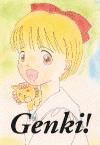\$B!Z4{4)![(J\$B!L:_8KL5!M(J \$B=i\$NI1\$A\$c\$sK\\$G\$9!#(J
\$B%*%U%;(J A5 100\$B%Z!<%8(J (\$B=iHG(J1995/7/30)
\$BEv=i!"2F%3%_\$K8~\$1\$FI1\$A\$c\$sK\\$r4k2hCf\$K!"(J \$BI1\$A\$c\$s\$NCB@8F|(J 7/30 \$B\$K!VI1\$A\$c\$s\$N%\$%Y%s%H!W\$,\$"\$k\$3\$H\$rCN\$j!"(J \$B\$=\$N%\$%Y%s%H\$K9g\$;\$?K\\$G\$9!#(J

\$BFbMF\$O!"\$^\$s\$,(J+\$BJ8>O(J+etc \$B\$GB?:L\$G\$9!#0&\$,\$3\$b\$C\$F\$^\$9(J(^^;;
{\$B\$9\$F\$-(J|\$B\$*\$7\$c\$l(J|\$B855\$(J}\$B\$J>n\$N\$"\$d\$7\$\$5-;v\$b\$"\$j\$^\$9(J:-)

SIDE A:
\$B\$^\$s\$,(J
• \$B\$"\$N\$M(J... by \$B\$(\$s\$H\$m\$T\$#(J,
• A day of Magical Land by \$B>.NS(J \$B\$Z\$k\$b(J,
• HIMEKO THE MAGICAL PRINCESS by \$B\$d\$@%b%s(J
• \$B\$O\$@\$7\$N%l%s(J by \$B&M(J2
• \$B\$*\$^\$1\$NI1\$A\$c\$s(J by Y'SeeNa.
• \$BIwN)\$U\$o\$b\$3F|5-(J by \$B\$j\$k\$U\$#(J
\$B>.@b(J
• \$BLL1F(J by \$B;V2l(J \$B2B0T(J
\$B:BCL2q(J
• \$B%7%M%^(JCD \$B!A(J FIVE YEARS LATER \$B!A(J \$B:BCL2q(J by \$BIZ;N(J,\$B>.;3(J,\$B>.ED(J,\$BLpED(J
\$B%\$%i%9%H(J,\$B%U%j!<%H!<%/(J, \$B%3%i%`(J
• \$B%;!<%i!\$B%\$%i%9%H(J by \$B\$9\$(\$T\$g\$s(J
• \$B%(%j%+MM\$rC5\$;(J by \$B\$H\$\$(J
• \$B%\$%i%9%H(J by \$B%?%+\$O\$7(J
• \$B\$d\$C\$Q\$j=w\$N;R\$O2D0&\$/\$J\$/\$A\$c\$M(J by (\$B\$G(J)
• \$B!VI1\$A\$c\$s\$N%j%\%s!W%0%C%:(J by \$B?e>e(J \$B7CB@(J
• August Aphrodite by \$B?eBt(J \$BIqF`(J
• \$B%(%W%m%s0&H~\$N\$*855\$%l%7%T(J by \$B\$R\$`\$+(J
\$BHb3((J: \$B\$R\$`\$+(J
\$BI=;f(J: \$B\$7\$g\$&\$A\$c\$s(J
SIDE B:
\$BO@J8(J
Short Story
Report
Dictionary
Column
• \$BI1\$A\$c\$s\$N%0%C%:\$N\$h\$&\$J\$b\$N(J by \$B?e>e(J \$B7CB@(J
\$B>R2p(J
\$BN"Hb3((J: \$B\$(\$s\$H\$m\$T\$#(J
\$BN"I=;f(J: \$B\$7\$g\$&\$A\$c\$s(J

#### Oshare! \$B!](J Hanepen Project 2 \$B!](J\$B!Z4{4)![(J 2\$BHVL\\$NI1\$A\$c\$sK\\$G\$9!#(J
\$B%*%U%;(J A5 44\$B%Z!<%8(J (\$B=iHG(J1995/12/25)
\$BNc\$K\$h\$C\$F!"1&\$+\$iFI\$`(J SIDE A \$B\$H:8\$+\$iFI\$`(J SIDE B \$B\$H\$\$\$&9=@.\$G!"(J SIDE A \$B\$N\$^\$s\$,\$O!"?4CH\$^\$kOC(J, \$B%.%c%0Ey\$H%P%i%(%F%#\$KIY\$s\$G\$^\$9!#(J \$B:#2s(JSIDE B \$B\$O>/\$J\$\$\$G\$9\$,(J \$B!V\$_\$s\$J\$G(J \$B\$\$\$1\$\$\$1%4!<%4!<\$8\$c!A\$s\$W\$C!W(J\$B\$d(J \$B!V?eBt@h@8%5%\$%s2q%l%]!<%H!WEy\$N5-;v\$,\$"\$j\$^\$9!#(J

SIDE A:
\$B\$^\$s\$,(J
• \$B\$"\$J\$?\$,K5\$K\$\$\$F\$/\$l\$?(J by \$B\$(\$s\$H\$m\$T\$#(J
• \$B\$7\$g\$;\$s=nL1\$O\$=\$s\$J\$b\$N(J by \$B\$Z\$k\$b(J
• \$B%j%\%s\$O\$_\$s\$J\$N0Y\$K(J by \$B\$d\$@%b%s(J
• \$B%-%9(J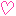\$B%-%9(J\$B88A[6J(J(\$B%U%!%s%?%8%"(J) by \$B\$d\$@%b%s(J
• 4\$B%3%^\$^\$s\$,(J by \$B&M(J2
• \$B@D=UKbK!%3%a%G%#I1\$A\$c\$s\$N!D(J by \$B\$Z\$k\$b(J
\$B;m(J,\$B%(%C%;%\$(J
• \$B=%1+(J by \$B;V2l(J \$B2B0T(J
• \$BIwN)%N!<%H(J by R.Goto
\$B%\$%i%9%H(J
• St.\$BI1\$A\$c\$s(Jby Y'SeeNa.
• \$BIqF'2q(J by \$B\$Z\$k\$b(J
• \$BI1\$A\$c\$s\$N%9%4%m%/(J by \$B\$d\$@%b%s(J
• \$BI1\$A\$c\$s\$N%a%m%G%#(J by \$B\$9\$(\$T\$g\$s(J
• \$B%\$%i%9%H(J by \$B%?%+\$O\$7(J
\$BHb3((J: \$B\$d\$@%b%s(J
\$BI=;f(J: \$B\$7\$g\$&\$A\$c\$s(J
SIDE B:
\$B%\$%i%9%H(J
• \$B0&H~(J by \$B\$7\$g\$&\$A\$c\$s(J
• \$B\$U\$?\$j\$N6&DLE@\$O2?\$G\$7\$g\$&!)(J by Y'SeeNa.
Report
• \$B\$j\$\\$sAO4)(J40\$B<~G/5-G0%5%\$%s2q(Jat\$BEl5~%l%](J by \$B0&LnH~F`;R(J
\$B2r@b(J
\$B>R2p(J
\$BN"Hb3((J: \$B\$Z\$k\$b(J
\$BN"I=;f(J: \$B\$7\$g\$&\$A\$c\$s(J

#### Suteki! \$B!](J Hanepen Project 3 \$B!](J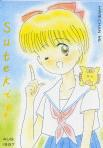\$B!Z?74)![%7%j!<%::G8e\$H\$J\$kI1\$A\$c\$sK\\$G\$9!#(J
\$B%*%U%;(J A5 68\$B%Z!<%8(J (\$B=iHG(J1997/08/17)
\$B\$\$\$D\$b\$I\$*\$j!"I=\$+\$iFI\$`(J SIDE A \$B\$HN"\$+\$iFI\$`(J SIDE B \$B\$K\$h\$k9=@.\$G\$9!#(J
\$B\$?\$/\$5\$s\$N<9I.P(J)\$B!#(J \$BI1\$A\$c\$s!"\$a\$0\$?\$s%U%!%s\$J\$iGc\$C\$FB;\$OL5\$\$\$O\$:!#(J(\$B\$=\$3\$^\$G\$f!<\$+!d\$o\$?\$7(J)

SIDE A:
\$B\$^\$s\$,(J
• \$B\$b\$&0l?M\$NI1;R(J by \$B\$(\$s\$H\$m\$T\$#(J
• \$B%j%\%s3WL?%R%a%3(J by \$B\$d\$@%b%s(J
• \$B7/5n\$j\$78e(J by \$B>.NS\$Z\$k\$b(J
• \$B>/=w3WL?%R%a%3(J by \$B8rLnB@O:(J
• \$BI1\$A\$c\$s\$O\$*G/:"(J by \$B\$7\$g\$&\$A\$c\$s(J
• \$B\$^\$s\$,(J by \$B\$&\$A\$@\$h\$7\$?\$+(J
• \$BI1\$A\$c\$s\$H0{\$b\$&\$h!*(J by \$B\$(\$s\$H\$m\$T\$#(J
\$B;m(J
• \$B%(%j%+(J by \$B^{@P%?%i\$A\$c\$s(J
• \$B%+%5\$K\$^\$D\$o\$k%(%H%;%H%i(J \$B!](J \$B1+;1%o%k%D(J \$B!](J by \$B0&LnH~F`;R(J
\$B>.@b(J
• \$BCB@8F|\$K@@\$C\$?\$3\$H(J by \$BE7G7
\$B%\$%i%9%H(J/\$B%U%j!<%H!<%/(J
• \$BI1\$A\$c\$s\$H%]%3B@(J by \$B\$^\$j\$N\$+\$l\$s(J
• \$BI1\$A\$c\$s(J by \$B\$O\$i\$X\$j(J
• \$B0&H~\$A\$c\$s(J by \$BCfED\$k\$_#S(J
• \$BI1\$A\$c\$s(J by \$B%?%+\$O\$7(J
• \$BI1\$A\$c\$s\$H\$R\$^\$o\$j(J by \$B>.NS=c(J
• Melody & Hachi \$B!](J \$B6u?'\$N%a%m%G%#(J \$B!](J by \$B\$7\$g\$&\$A\$c\$s(J
• momorin, \$B\$@\$"(J\$B!](J \$B%A%c%\$%`(J \$B!](J by \$B\$(\$s\$H\$m\$T\$#(J
• \$B\$Z\$3\$A\$c\$s\$C\$F!D!#!](J \$BE7;H\$?\$A\$N%F%#!<%?%\$%`(J \$B!](J by \$B\$(\$s\$H\$m\$T\$#(J
• 3\$BG/8e\$NI1\$A\$c\$s(J by \$B;V2l(J \$B2B0T(J
• \$B\$3\$3\$+\$i@h\$ON"B&\$+\$iFI\$s\$G\$M(J by \$B\$7\$g\$&\$A\$c\$s(J
\$BHb3((J: \$B>.NS\$Z\$k\$b(J
\$BI=;f(J: \$B\$7\$g\$&\$A\$c\$s(J
SIDE B:
\$BFC=8(J
• \$B\$^\$k\$4\$HI1\$A\$c\$s\$N%j%\%s3+H/ by \$B?eBt(J \$BIqF`(J
• \$BI1\$A\$c\$s\$N%j%\%sEY%A%'%C%/!*(J by \$B\$9\$^\$C\$/(J (\$BA^3((J:\$B\$7\$g\$&\$A\$c\$s(J)
Web \$B%P!<%8%g%s(J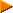\$BI1\$A\$c\$s\$N%j%\%sEY%A%'%C%/!*(J by \$B\$9\$^\$C\$/(J
\$B%\$%i%9%H(J
• CARDCAPTOR \$B\$R\$a\$3(J by \$B\$7\$g\$&\$A\$c\$s(J
• \$BI1\$A\$c\$s(J by \$B;V2l(J \$B2B0T(J
• Yukarin V by \$B\$R\$`\$+(J
\$BI1\$A\$c\$s\$N%j%\%s(JML\$B\$N\$4>R2p(J by \$B\$9\$^\$C\$/(J(\$BA^3((J:\$B\$7\$g\$&\$A\$c\$s(J) (Web \$B%P!<%8%g%s(J\$BI1\$A\$c\$s(JML\$B>R2p(J by \$B\$7\$g\$&\$A\$c\$s(J)
\$BN"Hb3((J: \$B\$O\$i\$X\$j(J
\$BN"I=;f(J: \$B\$7\$g\$&\$A\$c\$s(J

Hanepen: [ Top | Intro. | Hime-ML | What's New | ArtGallery | List ]
[ Shochan's Top ]

### Magical Extension

#### Magical Extension \$B!](J Hanepen Project 2.5 \$B!](J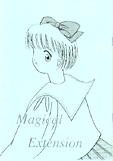\$B!Z4{4)![(J3\$BHVL\\$NI1\$A\$c\$sK\\$G\$9!#(J
\$B%3%T!<;o(J A5 20\$B%Z!<%8(J (\$B=iHG(J1996/10/20, 2\$BHG(J1996/12/29)
1996\$BG/(J10\$B7n\$N!VI1\$A\$c\$s\$N%\$%Y%s%H(JFINAL\$B!W\$"\$o\$;\$G=P\$7\$?K\\$G\$9!#(J
\$B\$[\$H\$s\$IJ,?HI1\$A\$c\$s%M%?\$NK\\$G\$9(J(^^;

\$B\$^\$s\$,(J
• \$B\$"\$k>/G/\$NC8\$\$Nx(J by \$B\$7\$g\$&\$A\$c\$s(J
\$B>.@b(J
• \$BM[1j(J by \$BJ8(J:\$B;V2l(J \$B2B0T(J, \$B3((J:\$B\$(\$s\$H\$m\$T\$#(J
\$B%\$%i%9%H(J
• \$BHb3((J \$B!](J Himechan's Ribbon! \$B!](J by \$B>.NS\$Z\$k\$b(J
• STREET FIGHTER II HIME by \$B\$d\$@%b%s(J
• HIME by \$B>.NS(J \$B=c(J
• \$B>.@b\$h\$j(J\$BJ8;R!&>>K\\$N?9B@O:=GIc\$5\$s\$N\$&\$A\$G(J. by \$B;V2l(J \$B2B0T(J
\$BI=;f(J: \$B\$7\$g\$&\$A\$c\$s(J

#### \$BKbK!3HD%(J Magical Extension 2 \$B!](J Hanepen Project 3.5 \$B!](J\$B!Z4{4)![(J5\$BHVL\\$NI1\$A\$c\$sK\\$G\$9!#(J
\$B%3%T!<;o(J B5 24\$B%Z!<%8(J (\$B=iHG(J1997/10/26)
1997\$BG/(J10\$B7n\$N!V\$^\$8\$+\$k!yI1\$A\$c\$s%D%"!

\$B\$^\$s\$,(J
• \$B;PKe\$N2qOC(J by \$B\$7\$g\$&\$A\$c\$s(J
• \$B%A%e%&0U0lICNx0l@8(J by \$B\$d\$@%b%s(J
• KISS KISS KISS by \$B>.NS\$Z\$k\$b(J
\$B%7%g!<%H(J
• \$B%(%j%+MM\$N%j%/%(%9%H3(F|5-(J by \$B^{@P%?%i\$A\$c\$s(J
\$BHb3((J: \$B\$d\$@%b%s(J
\$BN"I=;f(J: \$B>.NS\$Z\$k\$b(J
\$BI=;f(J: \$B\$7\$g\$&\$A\$c\$s(J

#### \$BKbK!3HD%(J Magical Extension 3 \$B!](J Hanepen Project 4.0 \$B!](J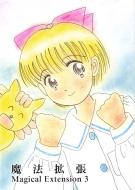6\$BHVL\\$NI1\$A\$c\$sK\\$G\$9!#(J
\$B=i\$N(J B5 \$B%*%U%;%C%H(J 56\$B%Z!<%8(J (\$B=iHG(J2000/4/2)
2000\$BG/(J4\$B7n(J2\$BF|\$N!V\$^\$8\$+\$k!yI1\$A\$c\$s#2(J(\$BIpB">.?y@n:j;TCf>.4k6H!&IX?M2q4[(J2F)\$B!W(J \$B\$"\$o\$;\$G=P\$7\$?K\\$G\$9!#(J

SIDE A:
Contents
• \$B\$^\$s\$,!?IwN)EEG>AH(J by \$B\$d\$@%b%s(J
• \$BI1\$A\$c\$s\$+\$k\$H%/%\$%:(J by \$B\$R\$a\$D\$&(J
• \$B\$^\$s\$,!?(J* Virgin Road * by \$B>.NS\$Z\$k\$b(J
• \$B%\$%i%9%H!?5'\$j(J by \$B^{@P%?%i\$A\$c\$s(J
• \$B>.@b!?\$1\$s\$+\$N8e\$NCgD>\$j(J by \$B\$G\$s\$9\$1(J (\$BA^3((J/\$B\$j\$k\$U\$#(J)
• \$B%\$%i%9%H!?0&;R\$*\$M\$(\$5\$s(J by \$BLn!986M3N\$I1(J
• \$B\$^\$s\$,!?\$^\$s\$,(J by \$B%O%k(J
• \$B%\$%i%9%H!?I1\$A\$c\$s(J by \$B%O%k(J
• \$B%\$%i%9%H!?I1\$A\$c\$s(J by \$B\$_\$A\$?\$s(J
• \$B\$^\$s\$,!?(JSpring \$B!](J \$B\$9\$W\$j\$s\$0(J \$B!](J by \$B\$j\$k\$U\$#(J
• \$B>.@b!?;D\$j9a(J by \$B;V2l2B0T(J (\$BA^3((J/\$B\$(\$s\$H\$m\$T\$#(J)
• \$B\$^\$s\$,!?(J \$B!](J \$B51\$-\$K\$R\$+\$l\$F(J \$B!](J by \$B\$(\$s\$H\$m\$T\$#(J
• \$B%\$%i%9%H!?I1\$A\$c\$s!u2V\$A\$c\$s(J by \$B\$^\$k\$A(J
\$BHb3((J: \$B>.NS\$Z\$k\$b(J
\$BI=;f(J: \$B\$7\$g\$&\$A\$c\$s(J
SIDE B:
Contents
• \$B%\$%i%9%H!?7k\$A\$c\$s(J by \$B\$7\$g\$&\$A\$c\$s(J
• \$B>W7b\$N;v\$B;m!?8a8e\$NM[\$@\$^\$j(J by \$BE7G7\$B%\$%i%9%H!?I1\$A\$c\$s(J by \$B\$j\$k\$U\$#(J
\$BN"Hb3((J: \$B\$d\$@%b%s(J
\$BN"I=;f(J: \$B\$7\$g\$&\$A\$c\$s(J

#### \$BKbK!3HD%(J Magical Extension 4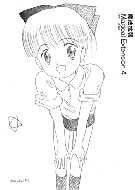7\$BHVL\\$NI1\$A\$c\$sK\\$G\$9!#(J
A5 \$B%3%T!<;o(J 16\$B%Z!<%8(J (\$B=iHG(J2002/10/13)
2002\$BG/(J10\$B7n(J13\$BF|\$N!VI1\$A\$c\$s\$N%j%\%s%"%K%a2=(J10\$B<~G/5-G0%*%s%j!<%\$%Y%s%H!W(J \$B\$"\$o\$;\$G=P\$7\$?K\\$G\$9!#(J

\$B\$b\$/\$8(J
• \$B\$^\$s\$,!?\$R\$a\$A\$c\$s\$N!D(J by \$B\$7\$g\$&\$A\$c\$s(J
• \$BI11X\$l\$]!<\$H(J by \$B\$7\$g\$&\$A\$c\$s(J
• \$BI1\$A\$c\$s\$N%j%\%s#M#LEj9F%"%I%l%9%i%s%-%s%0(J by \$B\$3\$d\$^\$h\$&\$\$\$A(J
• \$B\$^\$s\$,!?#4%3%^(J by \$B\$d\$@%b%s(J
• \$B\$^\$s\$,!?\$R\$a\$A\$c\$s\$H\$\$\$C\$7\$g(J by \$B\$7\$g\$&\$A\$c\$s(J
\$BI=;f(J: \$B\$7\$g\$&\$A\$c\$s(J

#### \$BKbK!3HD%(J Magical Extension 5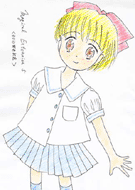\$B!Z?74)![(J8\$BHVL\\$NI1\$A\$c\$sK\\$G\$9!#(J
A5\$B%3%T!<;o(J 24\$B%Z!<%8(J (\$B=iHG(J2005/8/12)
2005\$BG/(J8\$B7n(J12\$BF|\$N2F%3%_\$"\$o\$;\$G=P\$7\$?K\\$G\$9!#(J

Contents
• \$B%\$%i%9%H!?I1\$A\$c\$s(J by HIMERIAN
• \$B\$^\$s\$,!?BgCO\$NLnK>(J by \$B\$d\$@%b%s(J
• \$B2r@b!?%i%0%J\$GI1\$A\$c\$s(J by \$B^{@P%?%i\$A\$c\$s(J
• \$BO@I>!?(J2005\$BG/\$K?eBt\$a\$0\$_\$r9M\$(\$k(J by \$B%?%+\$O\$7#R(J
• \$B%\$%i%9%H!?Nx\$9\$kH~>/=wF|HfLn\$R\$+\$k(J by \$B%U%j!<%I%j%C%R0KC+(J
• \$B>.@b!?Ic\$H1G2h\$HE_\$N1+(J by \$BE7Ln\$B%\$%i%9%H!?I1\$A\$c\$s(J by \$B%U%j!<%I%j%C%R0KC+(J
• \$B%\$%i%9%H!?I1\$A\$c\$s(J by \$B%m%s%2Kb?M#K(J
\$BI=;f(J: \$B\$7\$g\$&\$A\$c\$s(J
\$BN"I=;f(J: \$B\$(\$s\$H\$m\$T\$#(J

#### \$BKbK!3HD%(J Magical Extension 6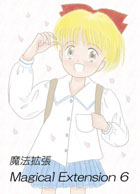\$B!Z?74)![(J9\$BHVL\\$NI1\$A\$c\$sK\\$G\$9!#(J
B5 \$B%*%U%;%C%H(J 32\$B%Z!<%8(J (\$B=iHG(J2006/4/2)
2006\$BG/(J4\$B7n(J2\$BF|\$NI1\$A\$c\$s%3%_%e%K%1!<%7%g%s\$"\$o\$;\$G=P\$7\$?K\\$G\$9!#(J
\$B=i\$a\$F\$N%G%8%?%kF~9F\$G\$9!#(J

Contents
• \$B%\$%i%9%H!?I1\$A\$c\$s(J by HIMERIAN
• \$B\$^\$s\$,!?\$:\$C\$H7/\$N\$3\$H\$_\$F\$-\$?\$+\$i\$M!D(J by \$B\$(\$s\$H\$m\$T\$#(J
• \$B\$^\$s\$,!?%]%3B@\$N%+%<(J by \$B\$7\$g\$&\$A\$c\$s(J
• \$B\$^\$s\$,!?BgCO!"K=Av%9%]%3B@\$N%+%<(J by \$B\$d\$@%b%s(J
• \$B\$^\$s\$,!?0&H~\$N?4G[;v(J by \$B\$^\$J\$?\$s(J
• \$B%\$%i%9%H!?%(%j%+!uI1\$A\$c\$s(J by \$B%O%k(J
• \$B%\$%i%9%H!?I1\$A\$c\$s(J by HIMERIAN
• \$B>.@b!?\$BI1\$A\$c\$s(JML\$B\$N>R2p(J by \$B\$7\$g\$&\$A\$c\$s(J
• \$BAGE(\$G\$*^/Mn\$JI1\$A\$c\$s(J by \$B>.NS\$8\$e\$s(J
\$BI=;f!&Hb3((J: \$B\$7\$g\$&\$A\$c\$s(J

Hanepen: [ Top | Intro. | Hime-ML | What's New | ArtGallery | List ]
[ Shochan's Top ]

### CG

#### \$BLC2[!VI1(J \$B\$E(J \$B\$/(J \$B\$7!W(J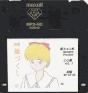\$B!Z4{4)![I1\$A\$c\$s#C#G=8(J volume.1
3.5\$B%\$%s%A%G%#%9%/(J 1.2 / 1.44 MB\$B!"(J(\$B=iHG(J1996/12/29)
\$B!V>oCsI1\$A\$c\$s(J [PC98, TOWNS, X11R5(Linux,SunOS,etc), Win16, Win32]\$B\$NA4%P!<%8%g%s!W\$H(J \$B!VI1\$A\$c\$s\$N(J KISS \$B%G!<%?!W\$N\$[\$\A4\$F\$r<}O?\$7\$F\$^\$9!#(J
\$B%a%\$%W%k%?%&%s(JBBS\$B\$NI1\$A\$c\$s%U%!%s\$N6(NO\$K46

```\$B"#%G%#%9%/FbMF(J  (\$B:nNN,!"=iHGH/9T=g\$K%=!<%H(J)
|
|- README.TXT ... \$B\$3\$N%I%-%e%a%s%H(J by \$B\$7\$g\$&\$A\$c\$s(J
|
|- RHIME ... \$B>oCsI1\$A\$c\$s(J
|   |          \$BI1\$A\$c\$s%U%!%s\$K\$O(J \$BDjHV\$H\$b8@\$(\$k%=%U%H!V>oCsI1\$A\$c\$s!W\$r(J
|   |          \$B3F5!oCsI1\$A\$c\$s(J PC98\$BHG(J Ver1.00  by MSP-Iris \$B!u(J \$B\$^\$k\$A(J
|   |   |                   98 DOS \$B\$GF0:n\$7\$^\$9!#(J
|   |   |
|   |   |- RHIME110.LZH ...\$B!J(JVer1.00->Ver1.10 \$B=\$@5:9J,!K(J by MSP-Iris \$B!u(J \$B\$^\$k\$A(J
|   |   |                   \$BBh(J2\$BOC7A<0(J \$B\$\$\$1\$\$\$1%4!<%4!<(J \$B\$rDI2C\$7\$^\$9!#(J
|   |   |                   bupdate \$B\$,I,MW\$G\$9!#(J
|   |   |
|   |   |- CRT_TOOL.LZH ... \$B>oCs%D!<%kI1\$A\$c\$sHG(J Ver1.00  by MSP-Iris
|   |                       CRT BIOS \$B%U%C%+!oCsI1\$A\$c\$s(J FM-TOWNS\$BHG(J Ver1.10 by \$B#M(Jalor
|   |                       \$B%?%&%s%:(J T-OS \$B\$N(J DOS \$B%3%s%=!<%k\$GF0:n\$7\$^\$9!#(J
|   |
|   |- UNIX ... Linux, SunOS4-5.x, HP-UX, IRIX, FreeBSD \$B\$J\$I(J
|   |   |
|   |   |- XHIME150.TGZ ... \$B>oCsI1\$A\$c\$s(J X Window\$BHG(J Ver1.50 by \$B\$7\$g\$&\$A\$c\$s(J
|   |                       \$B2rE`\$O(J "gzip -dc xhime150.tgz|tar xvf -" \$B\$G\$9!#(J
|   |                       \$BF0:n\$K\$O!"(JC compiler, X11R4 \$B0J>e\$,I,MW\$G\$9!#(J
|   |
|   |- WIN16
|   |   |- WH100   .LZH ... \$B>oCsI1\$A\$c\$s(J Windows(16bit)\$BHG(J Ver1.00 by \$B\$P\$s\$V!<(J
|   |                       Win3.1 \$BMQ(J  (1996-12-8 up\$BHG(J ^^)
|   |- WIN32
|       |- WH32100 .LZH ... \$B>oCsI1\$A\$c\$s(J Windows(32bit)\$BHG(J Ver1.00 by \$B\$P\$s\$V!<(J
|                           Win95, NT \$BMQ(J  (1996-12-8 up\$BHG(J ^^)
|
|
|- KISS ... \$BCe\$;\$+\$(%7%9%F%`(J(KISS)\$BMQ%G!<%?(J
|   |       \$B\$*;H\$\$\$N5!%a%\$%W%k\$NJ}!9(J
|   |                       \$B%P!<%8%g%s%"%C%WE}9gHG(J
|   |- HIMEKI_U
|   |   |- HIMEKI_U.LZH ... \$B\$2\$s\$-\$JI1\$A\$c\$s!JN"%P!<%8%g%s!K(J by \$B#U#Z#A#W#A(J
|   |   |
|   |   |- HIMEKI_1.LZH ... \$B\$2\$s\$-\$JI1\$A\$c\$s(J(\$B=\$@5%G!<%?(J \$BI=N"7sMQ(J) by \$B#U#Z#A#W#A(J
|   |                       \$B\$2\$s\$-\$JI1\$A\$c\$s%"%C%W%G!<%H\$G\$9!#(J
|   |                       HIMEKI_U.LZH \$B\$KBP\$7\$F%"%C%W%G!<%H\$7\$F\$/\$@\$5\$\$!#(J
|   |                       HIMEKISS.LZH \$B\$O%"%C%W%G!<%H:Q(J(\$B\$N\$O\$:(J)\$B\$G\$9!#(J
|   |
|   |- YUIKISS
|   |   |- YUIKISS .LZH ... \$B\$*\$7\$c\$^\$JL4\$A\$c\$s(J by \$B#U#Z#A#W#A(J
|   |
|   |- KS2HRCHI
|   |   |- KS2HRCHI.LZH ... \$BI1;R!u%(%j%+\$NCe\$;\$+\$(%G!<%?(J by \$B\$^\$k\$A(J
|   |                       \$BIwN)9b9;\$]\$\$@)I~\$H!"\$*\$^\$1(J \$BDI2C(J (see: KS2HRCHI.DOC)
|   |                       [\$B\$*\$^\$1\$O(J H \$B\$G\$9\$N\$GCm0U(J(^_^]
|   |- S_KSHIMA
|       |- S_KSHIMA.LZH ... \$BI1\$A\$c\$s(J&\$B%(%j%+\$5\$^\$C(J&\$BI1;R\$A\$c\$s(J by \$B\$7\$g\$&\$A\$c\$s(J
|                           \$B%^%8%+%k%W%j%s%;%9\$NI~(J \$BDI2CHG(J  Ver1.01a
|
|- CG
|- WAPI ... \$B?7IwN)%N\$o\$T(J
|   |- WPHIME01.LZH ... (PIC) \$B%Q%i%l%k%Q%i%l%kKe\$NL4\$A\$c\$s\$K\$J\$!!A\$C\$F\$k(J
|   |- WPHIME02.LZH ... (PIC) \$B\$(!\$BI1\$A\$c\$s(J/\$BI1\$E\$/\$7I=;f(J
|- S_HIMAF3.LZH ... (JPG) 3\$BG/8eI1\$A\$c\$s(J
|- S_YUI   .LZH ... (JPG) \$B6d0IJBLZ\$N7k\$A\$c\$s(J
```

Hanepen: [ Top | Intro. | Hime-ML | What's New | ArtGallery | List ]
[ Shochan's Top ]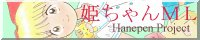Copyright (C) 1997-2007 Shochan-Nakayama, Hanepen Project, Himechan-ML
e-mail: shochan@tcp-ip.or.jp